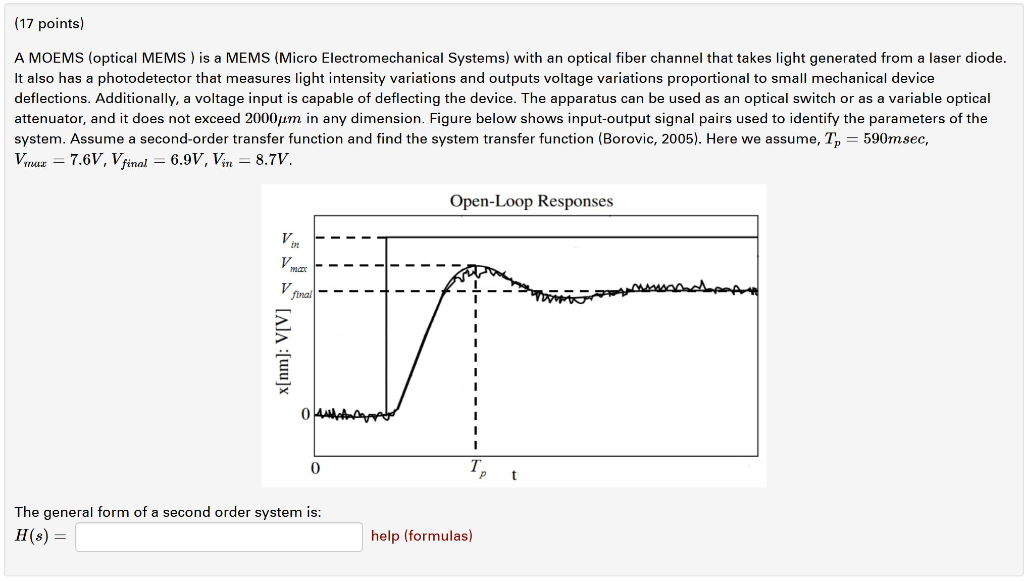Home / Answered Questions / Other / 17-points-a-moems-optical-mems-is-a-mems-micro-electromechanical-systems-with-an-optical-fiber-chann-aw799

# (Solved): (17 Points) A MOEMS (optical MEMS) Is A MEMS (Micro Electromechanical Systems) With An Optical Fiber...(17 points) A MOEMS (optical MEMS) is a MEMS (Micro Electromechanical Systems) with an optical fiber channel that takes light generated from a laser diode. It also has a photodetector that measures light intensity variations and outputs voltage variations proportional to small mechanical device deflections. Additionally, a voltage input is capable of deflecting the device. The apparatus can be used as an optical switch or as a variable optical attenuator, and it does not exceed 2000um in any dimension. Figure below shows input-output signal pairs used to identify the parameters of the system. Assume a second-order transfer function and find the system transfer function (Borovic, 2005). Here we assume, T, = 590msec, Vmax = 7.6V, V final = 6.9V, Vin = 8.7V. Open-Loop Responses A --- p ousantaransed - AA [u] - - - - ohnt - - - Tt The general form of a second order system is: H(8) = help (formulas)

We have an Answer from Expert